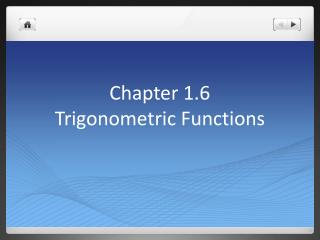# Chapter 1.6 Trigonometric Functions - PowerPoint PPT PresentationDownload PresentationChapter 1.6 Trigonometric Functions

Chapter 1.6 Trigonometric Functions
Download Presentation## Chapter 1.6 Trigonometric Functions

- - - - - - - - - - - - - - - - - - - - - - - - - - - E N D - - - - - - - - - - - - - - - - - - - - - - - - - - -
##### Presentation Transcript

1. Chapter 1.6 Trigonometric Functions

2. The Unit Circle

4. Examples • Examples • 1) 120° • 2) -45° • 3) 5π6 • 4) -3π2

5. Radian Measure • The RADIAN MEASURE of the angle ACB at the center of the unit circle equals the length of the arc that ACB cuts from the unit circle. Radius =1

6. Finding Arc Length • Find the length of an arc on a circle of radius 3 by a central angle of measure 2π/3. • S = r θ • = 3(2π/3) • = 2π

7. An Angle θ In Standard Position When an angle of measure θ is placed in standard position at the center of a circle of radius r, the six trigonometric functions of θ are defined as follows: sin θ = y/r cscθ = r/y Cos θ = x/r sec θ = r/x Tan θ = y/x cot θ = x/y

8. (SOHCAHTOA) • Sin – opp/hyp • Cos – adj/hyp • Tan – opp/adj • Csc – hyp/opp • Sec – hyp/adj • Cot – adj/opp

9. Graph of sin

10. Graph of cos

11. Graph of tan

12. Periodicity • Periodic Function, Period: A function f(x) is periodic if there is a postive number p such that f(x + p) = f(x) for every value of x. The smallest such value of p is the period of f.

13. Transformations of Trigonometric Graphs • Y = a f ( b ( x + c ) ) + d • A = vertical stretch or shrink/reflection about x-axis • B = horizontal stretch or shrink/ reflection about y-axis • C = Horizontal shift • D = vertical shift

14. Finding Angles in degrees and Radians • Find the measure of cos-1 (-0.5) in degrees and radians. • Put the calculator in degree mode and enter cos-1 (-0.5). You will get 120 degrees.

15. Using the Inverse Trigonometric Functions • Sinx = 0.7 • Take the sin-1 of both sides. • X = sin-1(0.7) • X = 0.775

16. Homework • Quick Review pg 52 # 1-4 • Section 1.6 Exercises pg 52 #1-10